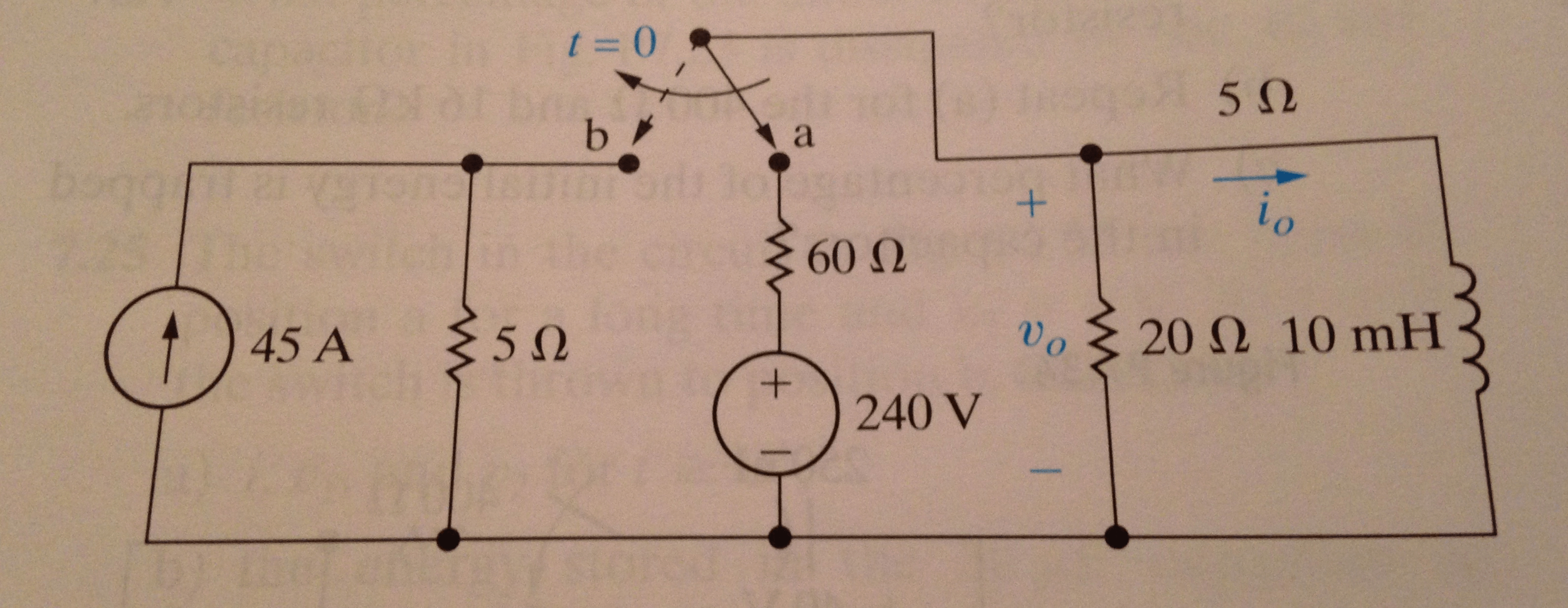# The switch in the circuit shown has been in position a for a long time. At t = 0, the switch moves instantaneously to position b.a) find the numerical expression for io(t) when t > = 0b) Find the numerical expression for vo(t) for t > = 0+.

The switch in the circuit shown has been in position a for a long time. At t = 0, the switch moves instantaneously to position b.

a) find the numerical expression for io(t) when t > 0

b) Find the numerical expression for vo(t) for t > 0+.This content is for Premium members only.